# RD Sharma Solutions for Class 10 Maths Chapter 8 Quadratic Equations Exercise 8.1

Degree 2 polynomials when equated to zero are called quadratic equations. These quadratic equations have certain conditions to satisfy in order to exist. Students build up strong basic knowledge by referring to RD Sharma Solutions Class 10, which are prepared by subject experts at BYJU’S. Also, the RD Sharma Solutions for Class 10 Maths Chapter 8 Quadratic Equations Exercise 8.1 PDF provided below is available for detailed references.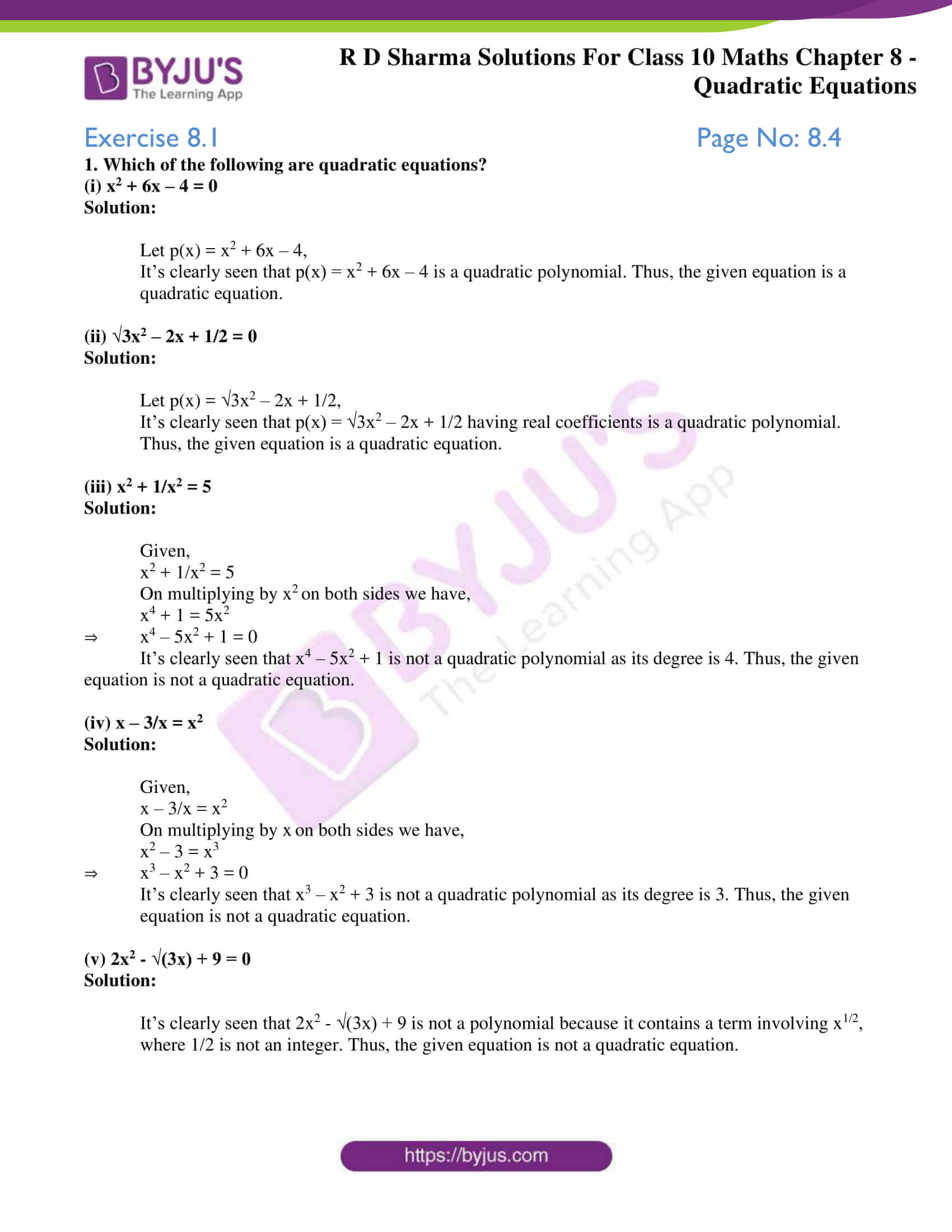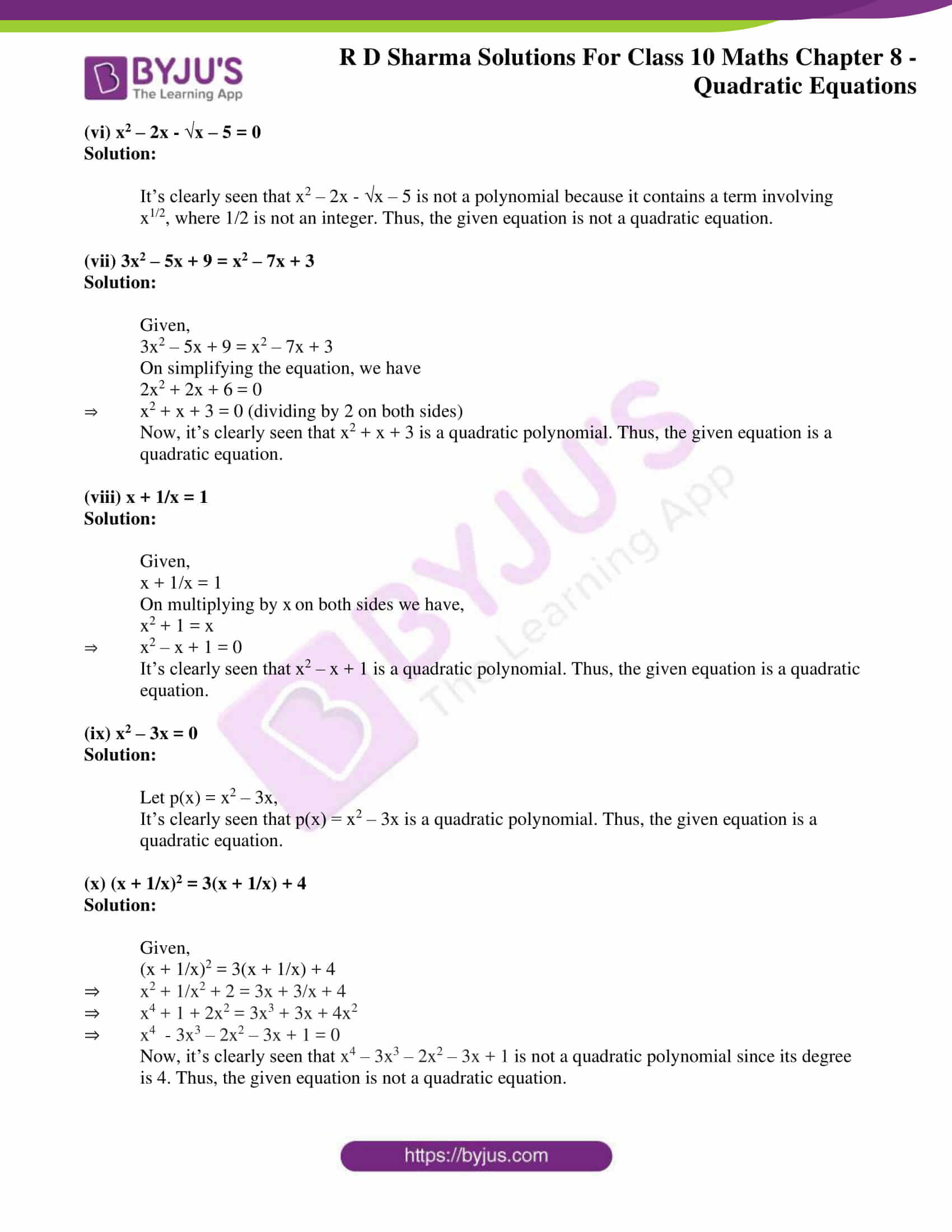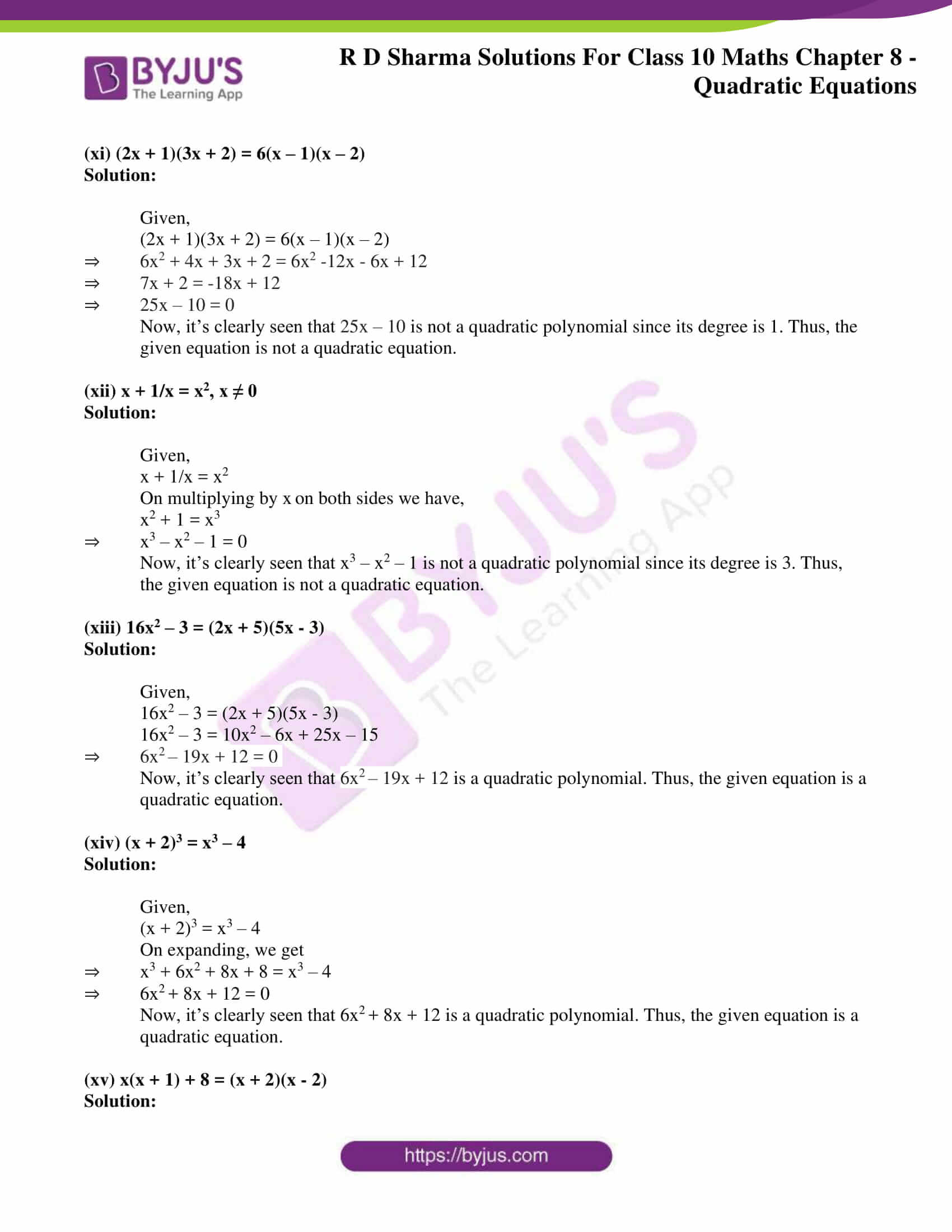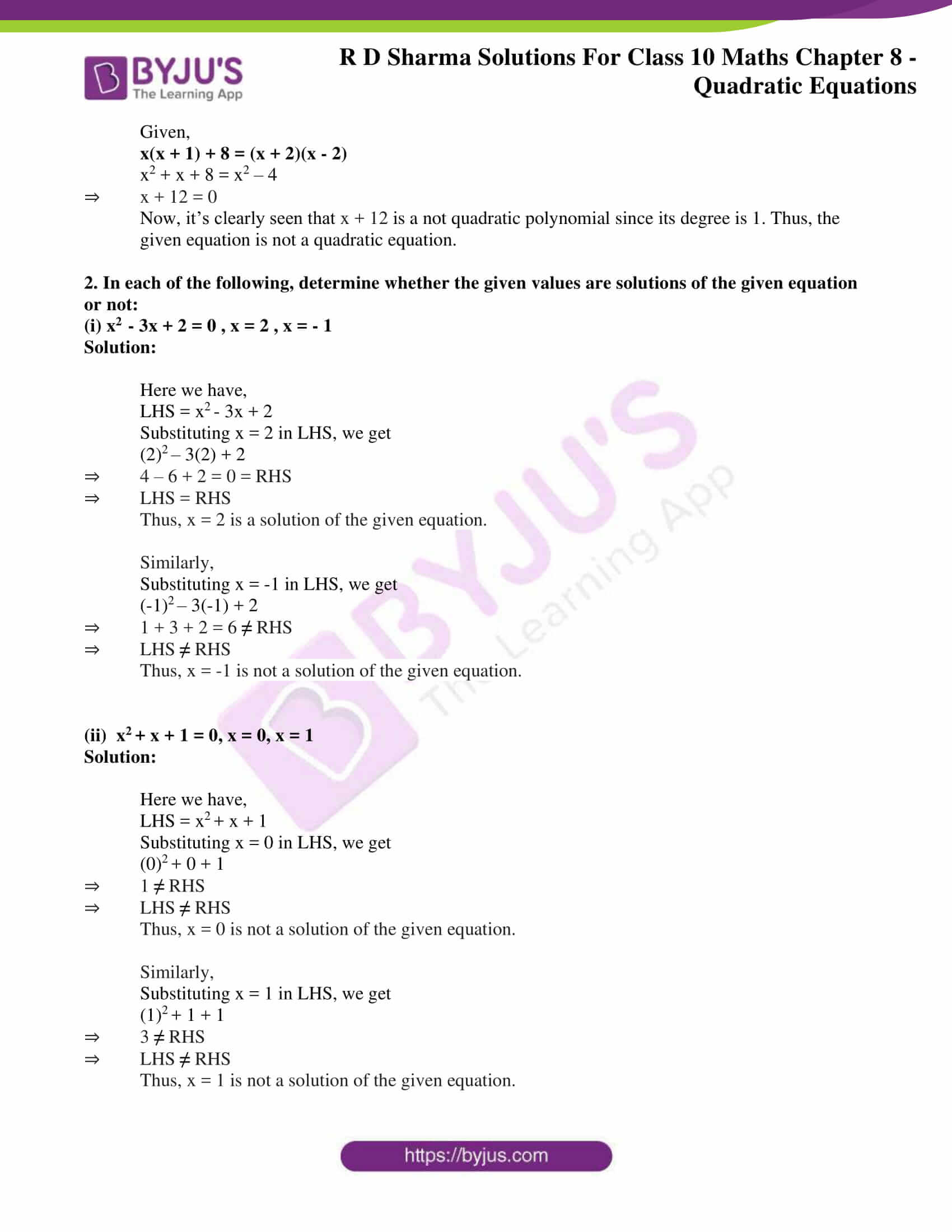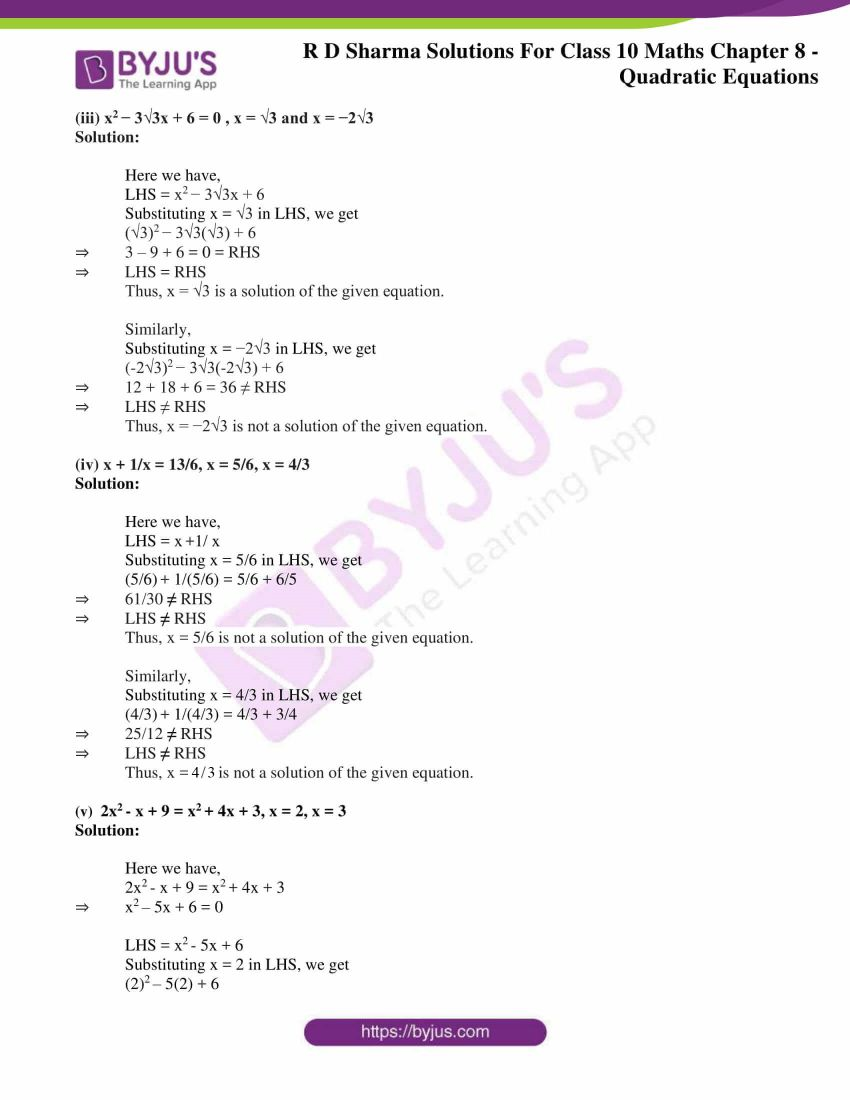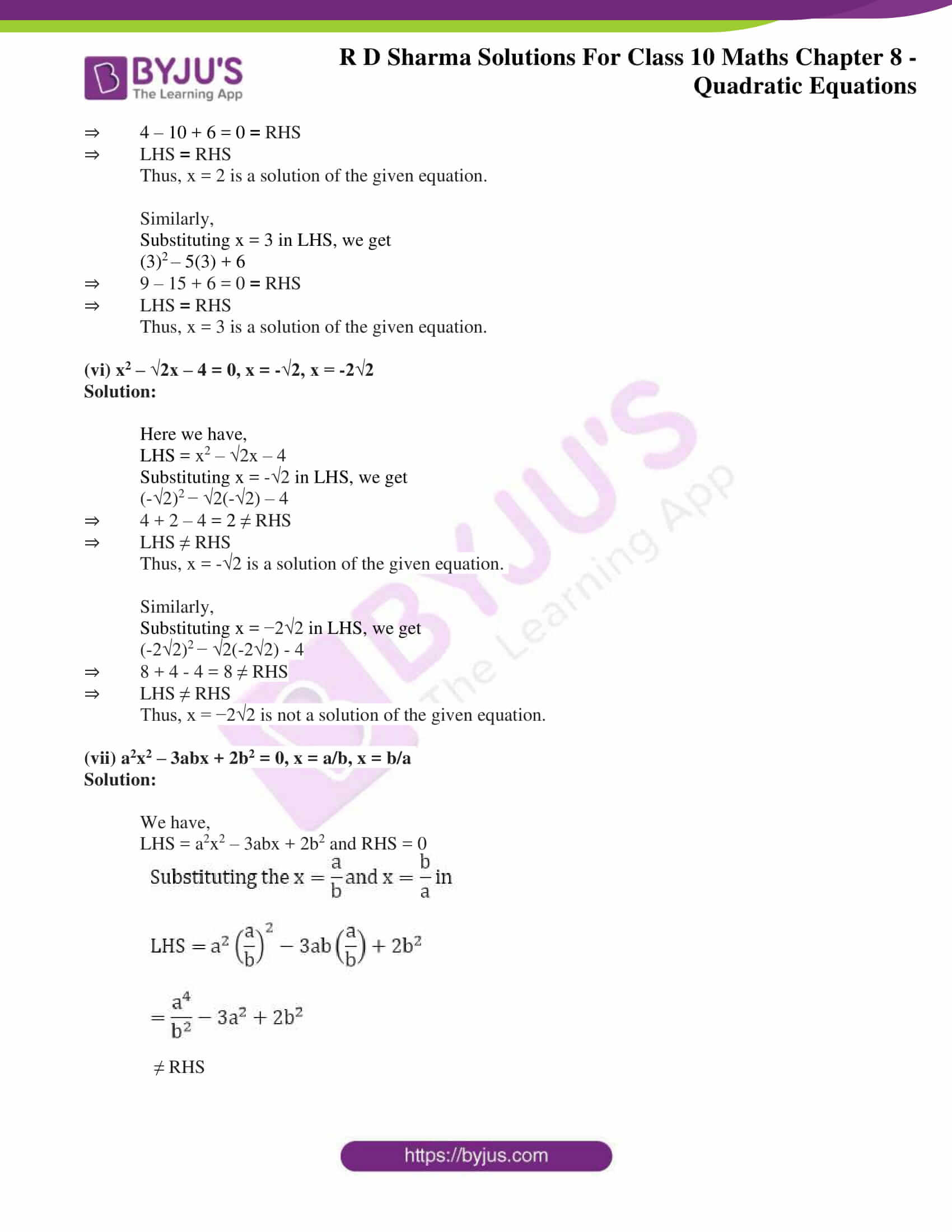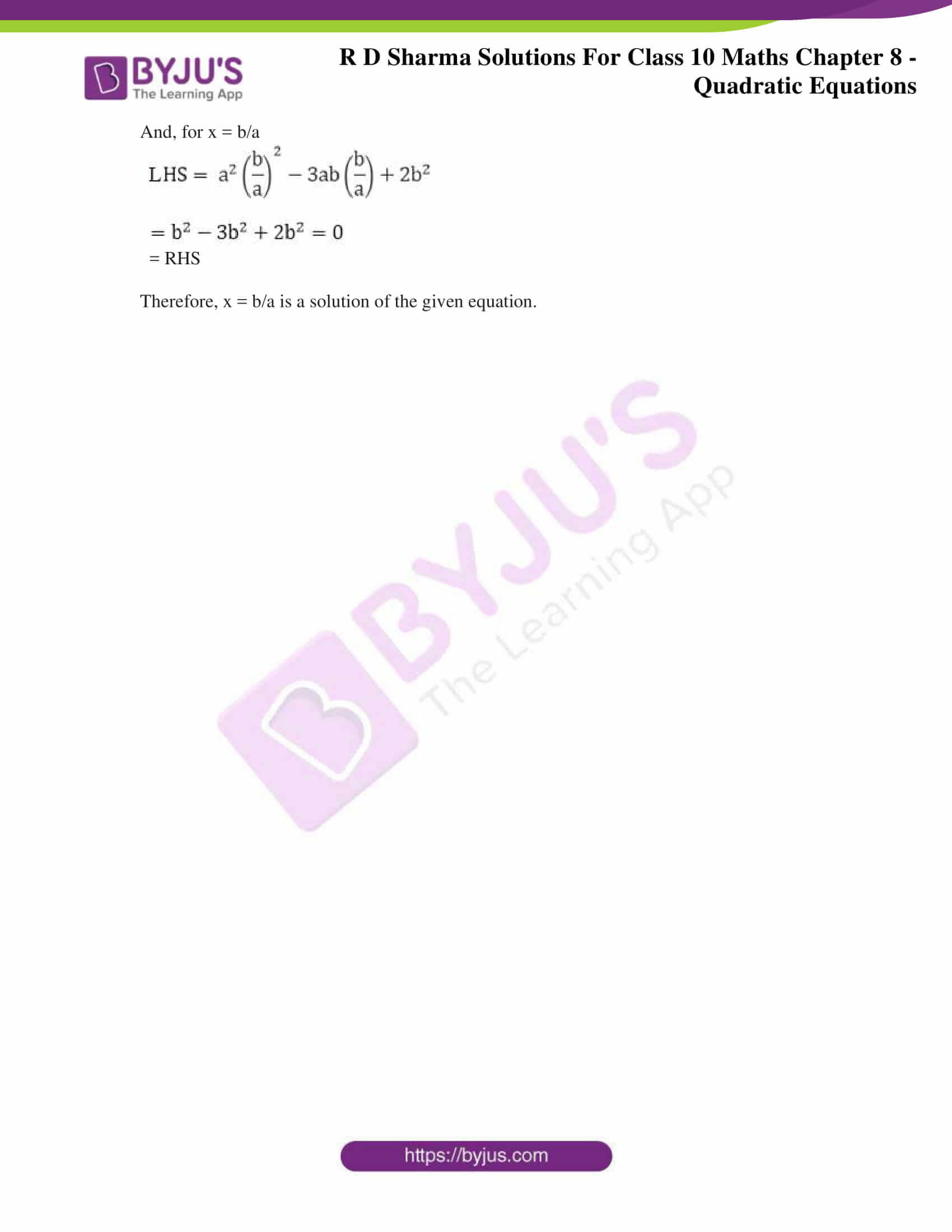### Access RD Sharma Solutions for Class 10 Maths Chapter 8 Quadratic Equations Exercise 8.1

1. Which of the following are quadratic equations?

(i) x2 + 6x – 4 = 0

Solution:

Let p(x) = x2 + 6x – 4,

It’s clearly seen that p(x) = x2 + 6x – 4 is a quadratic polynomial. Thus, the given equation is a quadratic equation.

(ii) √3x2 – 2x + 1/2 = 0

Solution:

Let p(x) = √3x2 – 2x + 1/2,

It’s clearly seen that p(x) = √3x2 – 2x + 1/2 having real coefficients is a quadratic polynomial. Thus, the given equation is a quadratic equation.

(iii) x2 + 1/x2 = 5

Solution:

Given,

x2 + 1/x2 = 5

On multiplying by x2 on both sides we have,

x4 + 1 = 5x2

⇒ x4 – 5x2 + 1 = 0

It’s clearly seen that x4 – 5x2 + 1 is not a quadratic polynomial as its degree is 4. Thus, the given equation is not a quadratic equation.

(iv) x – 3/x = x2

Solution:

Given,

x – 3/x = x2

On multiplying by x on both sides we have,

x2 – 3 = x3

⇒ x3 – x2 + 3 = 0

It’s clearly seen that x3 – x2 + 3 is not a quadratic polynomial as its degree is 3. Thus, the given equation is not a quadratic equation.

(v) 2x2 – √(3x) + 9 = 0

Solution:

It’s clearly seen that 2x2 – √(3x) + 9 is not a polynomial because it contains a term involving x1/2, where 1/2 is not an integer. Thus, the given equation is not a quadratic equation.

(vi) x2 – 2x – √x – 5 = 0

Solution:

It’s clearly seen that x2 – 2x – √x – 5 is not a polynomial because it contains a term involving x1/2, where 1/2 is not an integer. Thus, the given equation is not a quadratic equation.

(vii) 3x2 – 5x + 9 = x2 – 7x + 3

Solution:

Given,

3x2 – 5x + 9 = x2 – 7x + 3

On simplifying the equation, we have

2x2 + 2x + 6 = 0

⇒ x2 + x + 3 = 0 (dividing by 2 on both sides)

Now, it’s clearly seen that x2 + x + 3 is a quadratic polynomial. Thus, the given equation is a quadratic equation.

(viii) x + 1/x = 1

Solution:

Given,

x + 1/x = 1

On multiplying by x on both sides we have,

x2 + 1 = x

⇒ x2 – x + 1 = 0

It’s clearly seen that x2 – x + 1 is a quadratic polynomial. Thus, the given equation is a quadratic equation.

(ix) x2 – 3x = 0

Solution:

Let p(x) = x2 – 3x,

It’s clearly seen that p(x) = x2 – 3x is a quadratic polynomial. Thus, the given equation is a quadratic equation.

(x) (x + 1/x)2 = 3(x + 1/x) + 4

Solution:

Given,

(x + 1/x)2 = 3(x + 1/x) + 4

⇒ x2 + 1/x2 + 2 = 3x + 3/x + 4

⇒ x4 + 1 + 2x2 = 3x3 + 3x + 4x2

⇒ x4 – 3x3 – 2x2 – 3x + 1 = 0

Now, it’s clearly seen that x4 – 3x3 – 2x2 – 3x + 1 is not a quadratic polynomial since its degree is 4. Thus, the given equation is not a quadratic equation.

(xi) (2x + 1)(3x + 2) = 6(x – 1)(x – 2)

Solution:

Given,

(2x + 1)(3x + 2) = 6(x – 1)(x – 2)

⇒ 6x2 + 4x + 3x + 2 = 6x2 -12x – 6x + 12

⇒ 7x + 2 = -18x + 12

⇒ 25x – 10 = 0

Now, it’s clearly seen that 25x – 10 is not a quadratic polynomial since its degree is 1. Thus, the given equation is not a quadratic equation.

(xii) x + 1/x = x2, x ≠ 0

Solution:

Given,

x + 1/x = x2

On multiplying by x on both sides we have,

x2 + 1 = x3

⇒ x3 – x2 – 1 = 0

Now, it’s clearly seen that x3 – x2 – 1 is not a quadratic polynomial since its degree is 3. Thus, the given equation is not a quadratic equation.

(xiii) 16x2 – 3 = (2x + 5)(5x – 3)

Solution:

Given,

16x2 – 3 = (2x + 5)(5x – 3)

16x2 – 3 = 10x2 – 6x + 25x – 15

⇒ 6x2 – 19x + 12 = 0

Now, it’s clearly seen that 6x2 – 19x + 12 is a quadratic polynomial. Thus, the given equation is a quadratic equation.

(xiv) (x + 2)3 = x3 – 4

Solution:

Given,

(x + 2)3 = x3 – 4

On expanding, we get

⇒ x3 + 6x2 + 8x + 8 = x3 – 4

⇒ 6x2 + 8x + 12 = 0

Now, it’s clearly seen that 6x2 + 8x + 12 is a quadratic polynomial. Thus, the given equation is a quadratic equation.

(xv) x(x + 1) + 8 = (x + 2)(x – 2)

Solution:

Given,

x(x + 1) + 8 = (x + 2)(x – 2)

x2 + x + 8 = x2 – 4

⇒ x + 12 = 0

Now, it’s clearly seen that x + 12 is a not quadratic polynomial since its degree is 1. Thus, the given equation is not a quadratic equation.

2. In each of the following, determine whether the given values are solutions of the given equation or not:

(i) x2  – 3x + 2 = 0 , x = 2 , x = – 1

Solution:

Here we have,

LHS = x– 3x + 2

Substituting x = 2 in LHS, we get

(2)– 3(2) + 2

⇒ 4 – 6 + 2 = 0 = RHS

⇒ LHS = RHS

Thus, x = 2 is a solution of the given equation.

Similarly,

Substituting x = -1 in LHS, we get

(-1)– 3(-1) + 2

⇒ 1 + 3 + 2 = 6 RHS

⇒ LHS RHS

Thus, x = -1 is not a solution of the given equation.

(ii)  x+ x + 1 = 0, x = 0, x = 1

Solution:

Here we have,

LHS = x+ x + 1

Substituting x = 0 in LHS, we get

(0)+ 0 + 1

⇒ 1 RHS

⇒ LHS RHS

Thus, x = 0 is not a solution of the given equation.

Similarly,

Substituting x = 1 in LHS, we get

(1)+ 1 + 1

⇒ 3 RHS

⇒ LHS RHS

Thus, x = 1 is not a solution of the given equation.

(iii) x2 − 3√3x + 6 = 0 , x = √3 and x = −2√3

Solution:

Here we have,

LHS = x2 − 3√3x + 6

Substituting x = √3 in LHS, we get

(√3)2 − 3√3(√3) + 6

⇒ 3 – 9 + 6 = 0 = RHS

⇒ LHS = RHS

Thus, x = √3 is a solution of the given equation.

Similarly,

Substituting x = −2√3 in LHS, we get

(-2√3)2 − 3√3(-2√3) + 6

⇒ 12 + 18 + 6 = 36 ≠ RHS

⇒ LHS ≠ RHS

Thus, x = −2√3 is not a solution of the given equation.

(iv) x + 1/x = 13/6, x = 5/6, x = 4/3

Solution:

Here we have,

LHS = x +1/ x

Substituting x = 5/6 in LHS, we get

(5/6) + 1/(5/6) = 5/6 + 6/5

⇒ 61/30 RHS

⇒ LHS RHS

Thus, x = 5/6 is not a solution of the given equation.

Similarly,

Substituting x = 4/3 in LHS, we get

(4/3) + 1/(4/3) = 4/3 + 3/4

⇒ 25/12 RHS

⇒ LHS RHS

Thus, x = 4/3 is not a solution of the given equation.

(v)  2x– x + 9 = x+ 4x + 3, x = 2, x = 3

Solution:

Here we have,

2x– x + 9 = x+ 4x + 3

⇒ x2 – 5x + 6 = 0

LHS = x– 5x + 6

Substituting x = 2 in LHS, we get

(2)– 5(2) + 6

⇒ 4 – 10 + 6 = 0 = RHS

⇒ LHS = RHS

Thus, x = 2 is a solution of the given equation.

Similarly,

Substituting x = 3 in LHS, we get

(3)– 5(3) + 6

⇒ 9 – 15 + 6 = 0 = RHS

⇒ LHS = RHS

Thus, x = 3 is a solution of the given equation.

(vi) x2 – √2x – 4 = 0, x = -√2, x = -2√2

Solution:

Here we have,

LHS = x2 – √2x – 4

Substituting x = -√2 in LHS, we get

(-√2)2 − √2(-√2) – 4

⇒ 4 + 2 – 4 = 2 ≠ RHS

⇒ LHS ≠ RHS

Thus, x = -√2 is a solution of the given equation.

Similarly,

Substituting x = −2√2 in LHS, we get

(-2√2)2 − √2(-2√2) – 4

⇒ 8 + 4 – 4 = 8 ≠ RHS

⇒ LHS ≠ RHS

Thus, x = −2√2 is not a solution of the given equation.

(vii) a2x2 – 3abx + 2b2 = 0, x = a/b, x = b/a

Solution:

We have,

LHS = a2x2 – 3abx + 2b2 and RHS = 0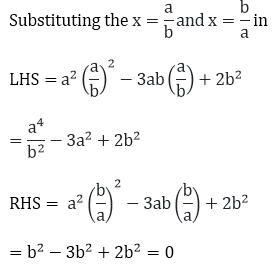≠ RHS

And, for x = b/a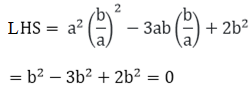= RHS

Therefore, x = b/a is a solution of the given equation.